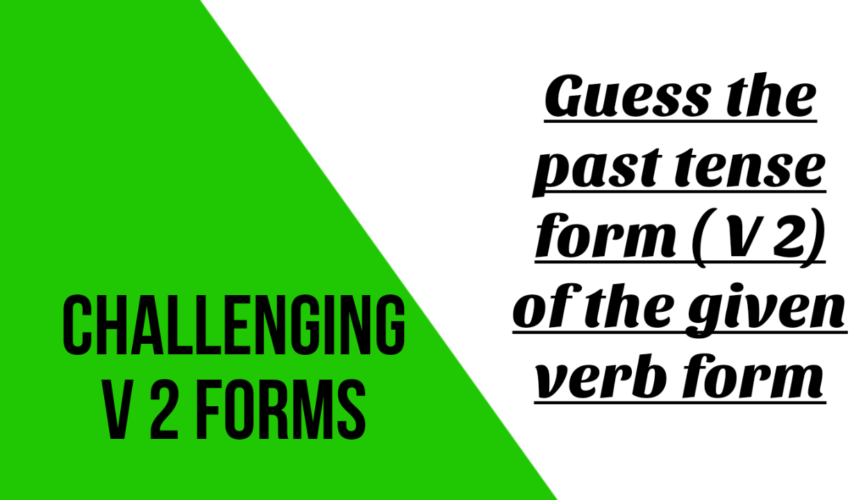11:50 pm Competitive Exam English

# Challenging past tense or V 2 form

In English some V 2 forms are confusing , here is the list of such confusing V 2 formGuess the past tense of the following verbs:

Present tense (V1) =   Past tense (V 2)

1. Grind  =
2. Feed    =
3. Cast    =
4. Deal   =
5. Forbid=
6. Forbear=
7. Rid  =
8. abide =
10. Kneel  =
11.  be       =
12. bid     =
14. cling
15. fling =
16. weep  =
17. sting   =
18. spin  =
19. strive =
20. swing =

Ans:
1. ground
2. fed
3. cast
4. dealt
6. forbore
7. rid
8. abode